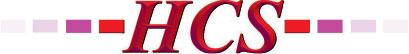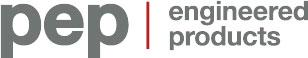AVENSYS.COMCANCOPPAS.COMCGIS.CAEVERESTAUTOMATION.COMFRANKLINEMPIRE.COMHCS1.COMPEP-PETRO.COMSWAGELOK.COMTHERMO-KINETICS.COMTHERMON.COMVANKO.NETVEGA.COMWAJAX.COMWESTECH-IND.COMWIKA.CA

## AutoQuiz: How to Measure Fluid Velocity Through a Pipe

The post AutoQuiz: How to Measure Fluid Velocity Through a Pipe first appeared on the ISA Interchange blog site.

AutoQuiz is edited by Joel Don, ISA’s social media community manager.

This automation industry quiz question comes from the ISA Certified Control Systems Technician (CCST) program. Certified Control System Technicians calibrate, document, troubleshoot, and repair/replace instrumentation for systems that measure and control level, temperature, pressure, flow, and other process variables. Click this link for more information about the CCST program.### A fluid is flowing through a 10-inch (0.254 m) diameter pipe at a velocity of 6 feet/sec (1.83 m/sec). When the pipe reduces to an 8-inch (0.2032 m) diameter, and all other flowing parameters remain the same, the fluid velocity becomes how many feet/sec (meters/sec).

a) 2.550 (0.77724)
b) 6.075 (1.85166)
c) 9.375 (2.8575)
d) 12.75 (3.8862)
e) none of the above

The “constant” between the run of pipe that has a 10-inch diameter and the run of pipe with a diameter of 8 inches is that the flow rate (and other fluid properties) is the same in both pipe lengths.

Flow through a round pipe can be expressed as:

Q = Velocity (ft/sec) x Area of Pipe (ft2 / m2)

Since flow is constant between the two pipe sizes, we can set:

Velocity1  x  Area1  =  Velocity2  x  Area2 ,

where the subscript = 1 for the initial conditions (10-inch pipe) and subscript = 2 for the final conditions (8-inch pipe).

Solving for Velocity2:

Velocity2   =  Velocity1  x  Area1 / Area2

For the 10-inch pipe, Area (in ft2) = pi x D2 / 4 = 0.5454 ft2.  (Don’t forget to divide 10 inches by 12 to get diameter in feet before “squaring.”)
Metric: A = pi x 0.2542 / 4 = 0.0506 m2

For the 8-inch pipe, Area (in ft2) = pi x D2 / 4 = 0.3490 ft2
Metric: A = pi x 0.20322 / 4 = 0.0324 m2

Substituting these values in the equation for Velocity2 above:

Velocity2   =  Velocity1  x  Area1 / Area2

Velocity2   =  6.0 ft/sec  x 0.5454 / 0.3490 =  9.375 ft/sec
Metric: V = 1.83 m/sec x  0.0506 / 0.0324 = 2.857 m/sec